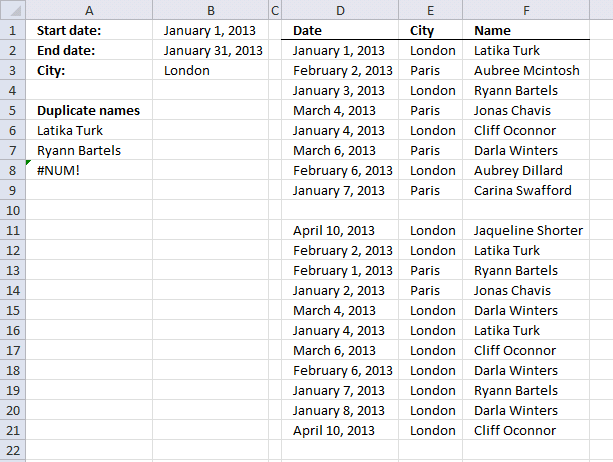Author: Oscar Cronquist Article last updated on August 24, 2017

The following worksheet allows you to search for duplicate names using a date range (cell B1 and B2) and a condition (cell B3).The result is displayed in cell A6 and below.

Array formula in cell A6:

=INDEX(\$F\$2:\$F\$21, SMALL(IFERROR(IF(MATCH(IF((\$D\$2:\$D\$21<=\$B\$2)*(\$D\$2:\$D\$21>=\$B\$1)*(\$E\$2:\$E\$21=\$B\$3),\$F\$2:\$F\$21,""), IF((\$D\$2:\$D\$21<=\$B\$2)*(\$D\$2:\$D\$21>=\$B\$1)*(\$E\$2:\$E\$21=\$B\$3), \$F\$2:\$F\$21,"A"), 0)<>MATCH(ROW(\$F\$2:\$F\$21), ROW(\$F\$2:\$F\$21)), MATCH(ROW(\$F\$2:\$F\$21), ROW(\$F\$2:\$F\$21))), ""), ROW(A1)))

Extract a list of alphabetically sorted duplicates from a column

The following array formula extracts duplicate values sorted from A to Z from cell range B3:B21. Excel array formula in […]

This post explains how to extract duplicate values using a condition:

Create a list of duplicates where adjacent cell value meets a condition

Question: How do I filter duplicates with a condition? Answer: Column B contains category and column C contains Items. Only […]

Filter a unique distinct list:

5 easy ways to extract Unique Distinct Values

First, let me explain the difference between unique values and unique distinct values, it is important you know the difference […]

Extract a list of duplicates:

Extract a list of duplicates from a column

The array formula in cell C2 extracts duplicate values from column A. Only one duplicate of each value is displayed […]

#### How to enter an array formula

1. Copy (Ctrl + c) above formula
2. Double click on cell A6
3. Paste (Ctrl + v) to cell A6
4. Press and hold CTRL + SHIFT simulatneously
5. Press Enter once
6. Release all keys

Your formula now looks like this: {=array_formula}
Don't enter the curly brackets, they appear automatically.